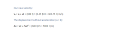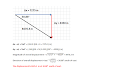Once you've learned the basics of modeling constant-acceleration motion, then what? Models that track moving objects are useful but very limited in their scope. There is much more to the world that we could hope to understand.*

And so we will expand our models of the world. And we shall start by asking,

For objects in motion, when and why does that motion change?

I'm going to present to you some data on a small spacecraft, currently traveling from the Earth toward Jupiter. I'm showing the spacecraft's velocity and acceleration, over time, which you're used to seeing. I'm also showing you the force (provided by the propulsion system) acting on the spacecraft. See if you can detect any patterns.

You might think that the best way to expand our models is to incorporate motion where the acceleration is NOT constant.

The reason we don't pursue this is because it requires calculus.

Furthermore, the topic is almost never covered in high school and often not even in college, even for physics majors.

*Can we understand the world without modeling it? Yes, at least many aspects of it. Modeling, however, leads to a deeper understanding. It also allows us to predict the future.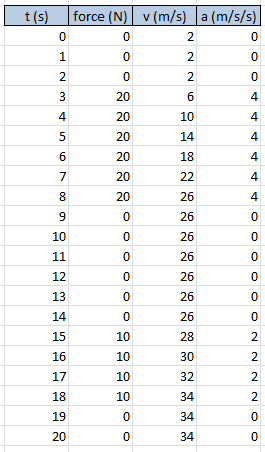I want you to spent a couple of minutes, right now, looking over the data table. Don't skip this part. Stop reading and do it.

• Can the spacecraft move without a force acting on it?
• What effect does the force have?

(The velocity and acceleration are relative to the Earth, by the way.)

Do you want a definition of force?

Conceptually, a force is a push or pull.

We'll define force mathematically soon.

In the case of our spacecraft, the force is a "push". The propulsion system of the spacecraft pushes it, periodically, toward Jupiter. I hope that you noticed, however, that a push is not required to maintain a velocity, at least for this spacecraft. If the propulsion system stops working after the 20 seconds described above, the spacecraft will "coast" toward Jupiter at a steady 34 m/s. It won't stop moving, relative to the Earth. It will stop accelerating. There's a big difference.

(Stop. Describe the relationship between force, velocity and acceleration without looking at your screen. If you can't do it, re-read and re-process this information.)Historically, people have struggled with the idea that something can move without being pushed.

And, of course, many things DON'T MOVE without being pushed.

But under the right circumstances, things CAN move without being pushed.

It took our species thousands of years to realize this.

Furthermore, if you'll recall our previous discussion of relative motion, you can change whether an object is moving or not simply by changing your perspective (reference frame).

You can't tell me that my couch is not moving because nothing is pushing on it. If I start walking away from it, it IS moving, relative to me.

This insight into the idea of relative motion guarantees that a force cannot be a prerequisite of motion.

Going deeper. If you dare.

Can my couch accelerate without a push (a force)?

It appears to accelerate if I accelerate away from it, right?

Yes, that's true. It does. However, we're building a model here. And long ago, people (beginning with Isaac Newton) thought hard about this idea and decided that the model is easier to manage if we require -- if we impose the rule -- that reference frames should not accelerate. Thus, we can say that in a non-accelerating reference frame (typically called an inertial reference frame), objects will not accelerate without a force acting on them.

Every once in a while, someone tries to model some small part of the universe and they don't realize that their reference frame is accelerating.

It makes it look like some object is accelerating without being pushed (or pulled).

Here's an example. If I slam on the brakes of my car, the bag in my passenger seat flies forward, actually accelerates forward (it seems to me), without being pushed.

This "force which does not exist" is called a fictitious force. It is a result of an accelerating reference frame.

We shall try to avoid accelerating reference frames. They are best left to the professionals, who understand what they're getting into. For us, we'll stick to inertial reference frames. Thus, objects will only accelerate when pushed or pulled; i.e. when there's a force on them.I'm going to present to you some data on a different spacecraft, currently traveling from the Earth toward Jupiter.

Compare it to the other one.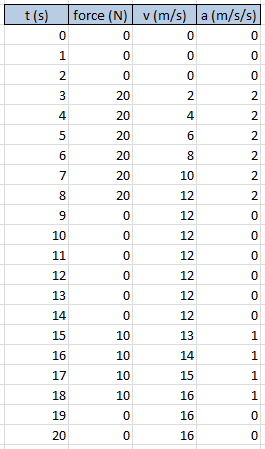The force (at each given moment) acting on this spacecraft is identical to the previous spacecraft, yet this spacecraft accelerates at only half the rate. What could cause this difference?

(By the way, the units for force, given as "N", will be discussed later. Don't worry about them for now.)

Imagine pushing equally hard on a toy car and a real car. Do they react identically?

Why is the toy car so much easier to accelerate?

Indeed, the important difference is in their masses. The larger the mass of the object, the less it will accelerate when you push on it.

This can be contrasted with the Anglican (Episcopal) and Catholic churches, for which the important difference is not in their masses.In summary,

we find (from data analysis) that the acceleration of an object is directly proportional to the force acting on it. If you double the force acting on some object, its acceleration will also double.

Furthermore,

we find (from data analysis) that the acceleration of an object is inversely proportional to the mass of the object. An object with twice the mass of another will experience half the acceleration, if the same force is applied to both.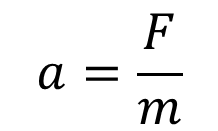And here we have one of the most important equations in physics. A simple relationship between three variables: force (F), mass (m), and acceleration (a).

Does the rate at which something accelerates depend upon only two things? Nothing else? Only force and mass? And the answer is ... yes, just two things. But realize that the force (its magnitude and direction) may itself depend upon other variables. The force with which I push a child on a swing set depends on how tired I am and whether I like the kid. And the force of the wind on the flag flying in front of my school depends on air temperature (and pressure) variation in the area. But while various things may affect the force, IF you are able to determine what that force is, and you have the object's mass, then you can easily find the object's acceleration. Yes, just two things.

Many books (and people) write this famous formula as F = ma. This is okay (they have my permission), but I prefer to write it as a fraction. Here's why:

F and m are both independent variables; they do not depend on a; rather, they determine a

is the dependent variable; the acceleration of some object depends on the object's mass and the force acting on that object, but neither F nor m depends on the a, nor does the F depend on the m, or vice versa

So I write the equation as a = F / m to emphasize that a is determined by F and m.

Take a look at the data tables above, for the two spacecraft, and determine the mass of each. (The values are not realistic, I admit.)

You should have found that the first spacecraft has a mass of 5 kg (yes, the units would be kilograms), while the second spacecraft has a mass of 10 kg.

UNITS

Let's discuss the units typically used for measuring mass and force.

(You already know the typical units for velocity (m/s) and acceleration (m/s2).)

In physics, force is almost always reported in units called "newtons", named after the great physicist Isaac Newton. Most people have no idea how strong a newton is. If I punch a wall with a force of 5 newtons, did I punch the wall hard? For me, it's the same with measuring temperature in Celsius. Is 40° C hot or cold? I have no idea. I'm an American.

It would be helpful for you to gain some intuitive sense for newtons as a unit of measure. But it's hardly required. It's just helpful. (Your best hope for doing this would be to work out the weight of various objects, in newtons. Didn't realize weight was a force? Your weight is a measure of how hard you're pushing against the scale. I'm tempted to explain this in more detail ... there's lots more detail ... but I'll save it for later.)

I'll give you three examples. A 150-pound man weights 668 newtons, on Earth. A 4000-pound car weighs 17,793 newtons, again on Earth. (They'd weigh less on the Moon.) And a gallon of milk weighs about 38 newtons. So, is 5 newtons very much?

Let's re-focus. Here is the proper definition of a newton.

A newton is the force required to accelerate a 1-kilogram mass at a rate of 1.0 m/s2.

Imagine having a 1-kilogram block of metal, sitting on a slippery (frictionless) table. It's at rest. How hard do you have to push on it, so that one second from now, it is moving at 1 m/s? That's how hard a newton is. And ... really ... it's not that hard.

Notice that the definition of a newton references a kilogram. For this reason, whenever you're measuring force in newtons, you must also be using kilograms to measure any and all masses. We must be consistent with our units!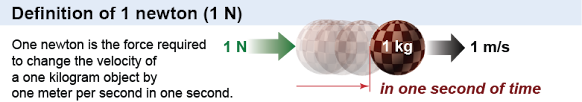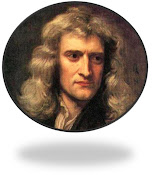As you probably can recognize, physicists prefer to work with SI units (aka the metric system).

Within this system of units, we have what are called base units, and we have derived units.

Derived units are defined in terms of base units. As an example, the newton (abbreviated as N) is a derived unit, defined as:

This can be read as an equation but also a definition. A newton (N) is equal to (or defined as) a kilogram (kg) times a meter (m) divided by seconds (s) squared. Kilograms, meters and seconds are all base units. (There are an additional 4 base units in the SI.) Note: The kilogram is the only base unit with a prefix. The base unit is the kilogram, not the gram.

*Don't confuse this m with the m for mass.

To aid your understanding, let's look at a couple of manipulations of this equation. Each of these manipulations is true.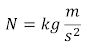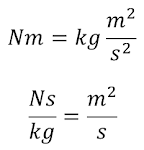If we know that a 25-kg mass has an acceleration of 3 m/s2, we can obtain the force that must be causing that acceleration.

Using F = ma,

F = (25 kg)(3 m/s2) = 75 kg m/s2

but since kg m/s2 is equivalent to N, we can write F = 75 N.

Likewise, a 10-kg mass with an acceleration of 4 m/s2 must be experiencing a force of 40 N.

But, remember, the force we are calculating here does not depend on the mass or acceleration. Rather, the acceleration depends on both the force and mass. Don't take F = ma to mean that F depends on m and a. It doesn't. That's why I prefer to write a = F / m.

Take a quick look at the data table to the left. Does it make sense? It should.

Does the data table to the right make sense?

(It shouldn't. Three rows contain mistakes. See 'em?)

Can a force be negative? Can acceleration? Can mass?

Have you ever seen a negative acceleration before?

And what does a negative acceleration mean?

I hope you remember that it does NOT mean something is slowing

down or decelerating. The negative sign is an indication of direction.

It's time to resurrect our notation for keeping track of direction for

vector quantities.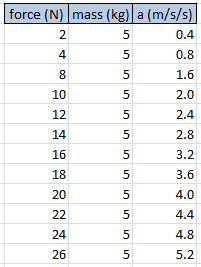If you want a definition of mass, you'll have to wait. I'll get to it eventually. For now, I'll hope you have a general idea of what it is.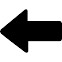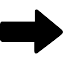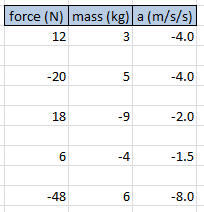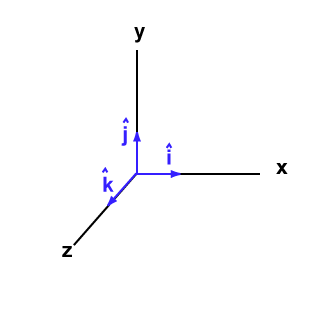When you're modeling some moving object, you must first choose where to station your Cartesian coordinate system, i.e. your axes. Once you've done that, any vector quantity that points "rightward" or "eastward" is said to be pointing in the i direction.

Likewise, the direction of vectors pointing "leftward" or "westward" is noted as -i. Furthermore, "up" and "northward" corresponds to j, and "down" and "southward" correspond to -j. If we had to work in 3-dimensions, we'd use k, but we've always confined ourselves to two dimensions.

Here is an equation from our past. It models something that is falling, somewhere on Earth.

You may also remember that, when modeling projectiles, I told you that I preferred to avoid the i and j notation and use ∆x and ∆y. Thus, the equation above might be written:

Well, we're bringing back the i and j notation. We do so because it is useful.

xf = (-4.896 j)t2 + 0.7678 j            (do you remember what the 0.7678 represents?)

∆y = -4.896t2 + 0.7678

Both acceleration and force are vector quantities. Thus, both can carry a negative sign.

Mass is not a vector quantity. It's a scalar. It has no direction and, therefore, cannot carry a negative sign. (I mean, negative mass? Really? What would that even mean?)

Take a look at the following (true) examples, in which I incorporate the direction of the vector quantities (as I should have been doing all along).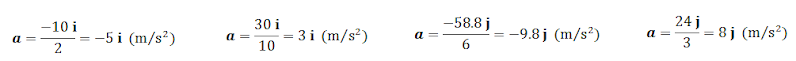I hope it's clear that the acceleration and force will always have the same direction.

I also want to remind you that objects don't always move in the direction of their acceleration. A car can be moving eastward and have a westward acceleration. Do you remember what this means?

It must then be true that objects don't always move in the direction of the force acting upon them. You already know of an excellent example. When you throw a ball upward, which direction is gravity (the force) acting? Can a ball move upward for several seconds, even as gravity is pulling downward on it? You bet. It happens all the time.

At the beginning of this page, I stated our current question of interest,

For objects in motion, when and why does that motion change?

And the answer... motion changes (things accelerate) when forces act. The only way the car in my driveway is going to change its motion (it's currently at rest) is for a force to act on it.

There are so many questions we could examine at this point. Have any of these crossed your mind?

• If I push on my house, it doesn't move. There's a force, so why no acceleration?
• How do you find the acceleration of something if multiple forces are acting on it all at once?
• How can a rocket move in space? There's nothing to push off.
• I can easily go from standing still to running. That's an acceleration, right? What force is acting on me when I begin to run?
• Is there a maximum force with which you can hit something?
• Why does everything on Earth fall at the same rate (-9.8 m/s2), ignoring air resistance?
• What is mass, exactly? (Interesting note: There is more than one type of mass.)
• What's the difference between mass and weight?

​We'll eventually tackle these questions, learning a lot in the process. But first we're going to practice incorporating force into our kinematic models, which we've spent so much time developing.

1. A 2,000-kg rocket is heading from the Earth to the Moon at a constant 600 m/s. The propulsion system is activated and a force of 500 N acts in the current direction of motion for 15 seconds. What is the displacement of the rocket during this period of time?

Try to visualize what is happening. Draw a picture if it helps. (Later, as problems become more complex, we will practice drawing such pictures.) You want to understand what is happening and what it is that you're trying to find. Don't jump too quickly to grabbing an equation and plugging numbers into it.

Clearly label the values stated in the problem statement. Include direction for vector quantities.

(As a coordinate system was not referenced, we choose to arrange our own. The obvious choice is to arrange our coordinate system with the x-axis connecting the Earth and Moon, with the unit vector i pointing toward the Moon.)

m = 2000 (kg) , vi = 600 i (m/s) , F = 500 i (N) , t = 15 (s)

We're trying to find ∆x.

What models can we use here? Certainly our new one that incorporates force: a = F / m.

From this model we'll work out the rocket's acceleration.

We also need a model that incorporates vi, t and ∆x

Let's use ∆x = vit + ½ at2.

We want to work out the value of ∆x; our model is already arranged to find it.

Let's solve for a:  a = 500 i / 2000 = 0.25 i (m/s2)

And now let's solve for ∆x∆x = (600 i)15 + ½(0.25 i)152 = 9028 i (m)

Our answer: The displacement of the rocket during the period of acceleration is 9028 meters toward the Moon.

Two additional values to work out:

-What is our new velocity?

-What would the displacement have been during this 15 seconds if the propulsion system had not been used?In words: "A force of 10 newtons to the west, acting on a mass of 2 kilograms, leads the mass to accelerate at 5 meters per second squared to the west."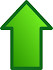Δx = vavgt               Δx = vit + ½ at2

vf = at + vi               vf2 = vi2 + 2aΔx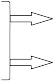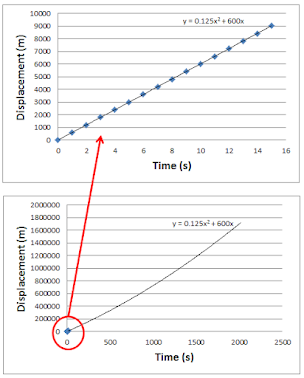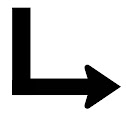We shouldn't go too long without looking at a graph.

Here, using the ∆x model (with t as a variable), I've plotted 15 data points showing how the displacement increases with time.

The graph looks linear, but it's not. It's parabolic ... obvious from the equation. In the second graph, I extended the trend line to show that it does actually curve.

Yes, as I'm sure you know, curved surfaces can look flat up close. For example, the Earth we're standing on.

2. A Boeing 737 aircraft (mass of 45,000 kg) is heading north at 265 m/s. A headwind (which, by definition, blows from directly in front of a plane, opposing its motion) exerts a constant force of 18,000 N for 2 minutes. If the pilot does not increase thrust to counter this headwind, what velocity would result? Furthermore, what is the displacement of the plane during this 2 minutes (still assuming the pilot does not correct for the slowing effect of the wind)?

I've visualized the problem. Have you?

Here's what we have: m = 45,000 (kg) , vi = 265 j (m/s) , F = -18,000 j (N) , t = 120 (s)

Here's what we want: vf = ? , ∆x = ?

This will require multiple models. We'll need a = F / m to obtain the acceleration. We'll need vf = at + vi to obtain our final velocity, and we'll need any of the other three kinematic models to find ∆x. (That's right, we can use any of the models that contain a ∆x! That's not always the case, but it works here because we'll have values for each of the variables in all three equations.)

a = -18,000 j / 45,000 = -0.40 j (m/s2)

vf = (-0.40 j)120 + 265 j = 217 j (m/s)

∆x = vavgt = ½(vi + vf)t = ½(265 j + 217 j)120 = 28,920 j (m)

∆x = vit + ½ at2 = (265 j)120 + ½(-0.40 j)1202 = 28,920 j (m)

vf2 = vi2 + 2aΔx leads to Δx = (vf2 - vi2) / 2a = ((217 j)2 - (265 j)2) / 2(-0.40 j) = 28,920 j (m)

The headwind results in a final velocity of 217 m/s to the north and a displacement of 28,920 m to the north.

3. The same Boeing 737 (mass of 45,000 kg) is now flying at 280 m/s at 30° south of east. A wind from the north (blowing directly southward) exerts a force of 15,000 N on the airplane. If the force lasts for 30 seconds, and the pilot does not adjust for it, what is the resulting velocity of the plane?

Our motion is 2-dimensional, and we must model it as so. Drawing vectors will help.At some point, you have to convert minutes to seconds, for the sake of unit consistency. I've gone ahead and done it.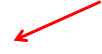I put "Displacement" and not "Position" on the vertical axis. That was intentional. Can you figure out why?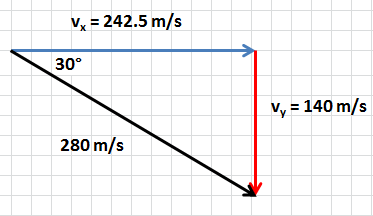I've represented the velocity as a vector (in black). I've also drawn in and calculated the x- and y- components (vx and vy) of the velocity.

As we are indicating direction with our i and j vectors, we update our two velocity components to read:

vx = 242.5 i (m/s)

vy = -140 j (m/s)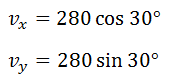The object is actually moving at 280 m/s, angled southeast (30° S of E, to be exact). Thinking of that motion as being comprised of two separate and independent velocities, one eastward and one southward, is a useful way of thinking of its motion. Its a useful model of what's happening. I've said it before, but make sure you understand this.

The force on the airplane is acting southward, so F = -15,000 j (N).

This results in an acceleration of:

a = F / m = -15,000 j / 45,000 = -0.333 j (m/s2)

This acceleration changes only the component of the velocity in the direction of -j. It has no effect on vx. (We saw the same thing when examining projectiles.)

Thus, 30 seconds of acceleration leads to a vertical velocity of vf = at + vi = (-0.333 j)30 + (-140 j) = -150 j (m/s).

After 30 seconds of wind, the plane is now flying southward at 150 m/s (instead of the previous 140 m/s) but is still flying eastward at 242.5 m/s.

The actual velocity of the plane is simply the vector sum of these two components. That is, "put them back together" to get the actual velocity (both magnitude and direction).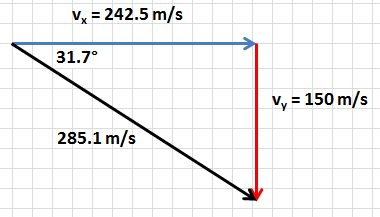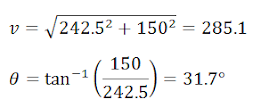We can state our answer now. As a result of the wind, the velocity is now 285.1 m/s at 31.7 south of east.

(You could have just said that the final velocity was 242.5 i - 150 j, but most teachers will require you to sum the vectors to prove that you can. Furthermore, you typically want to match the format of your answer to the format of the quantities presented in the problem.)

HEY...

Try to work out the displacement of the Boeing 737 during this 30 second period. DO IT! Get a pencil and paper and try it. Only AFTER you arrive at an answer, check your work here.

Realize that what we are doing here is exactly what we do for projectiles that are launched horizontally. Except for projectiles, we don't have to calculate the acceleration, because it's always -9.8 j (m/s2) on Earth.

The only difference in our approaches is that, for projectiles, I swapped the i and j notation for x and y. I did that because I found it less cumbersome. You might try it here, but I feel like the i and j notation will do a better job of keeping things straight.

practice  problemsa = F / m

The model or equation we worked out (through data analysis) originated in the mind of Isaac Newton, one of the most brilliant thinkers to have lived. Newton, born in England in 1642 (or 1643, depending on the calendar you use), identified this relationship among variables in 1687 in a book he titled Philosophiæ Naturalis Principia Mathematica (Mathematical Principles of Natural Philosophy). (Newton was English but wrote in Latin, a common practice among scientists at the time.)

If you were to examine a copy of this book, however, you would not find the equation. It was only much later that the words of Newton were recognized as equivalent to and recast as F = ma.

Here is what Newton actually said, from the 1729 English translation (from Latin) by Andrew Motte, based on Newton's 3rd edition, published three years earlier. (This is a photocopy from an actual copy of the book. The book itself, very old and very valuable, is kept at Wren Library at Trinity College, Cambridge. This is fitting, as Newton attended Trinity College as an undergraduate and later worked there.)

Well, at least we can find the word "force"! This Law II is interpreted as saying that an object's motion is changed when a force acts upon it, and the change takes place in the direction of the force. Furthermore, the larger the force, the larger the change.

You are forgiven for imagining that "alteration of motion" is a reference to acceleration. It isn't. It is now interpreted as a reference to the rate at which the object's momentum is changing. Ah, and we've yet to discuss momentum. It doesn't matter. Apparently, momentum and acceleration are related, and in the end, it comes out to F = ma.

By the way, there is debate as to whether this Law should be considered a definition of force. We're about to fall down a deep rabbit hole, so let's stop.

And yes, this is Newton's 2nd Law of Motion! And as you've probably heard, there are two others.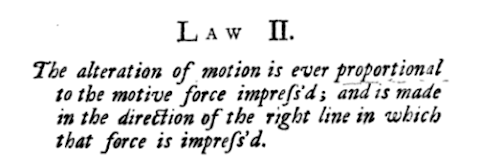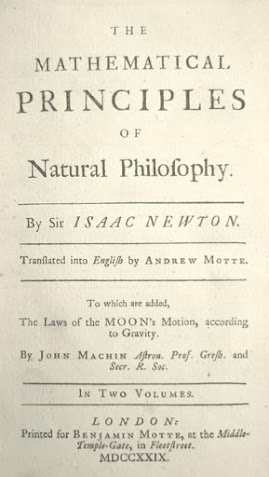MDCCXXIX = 1729